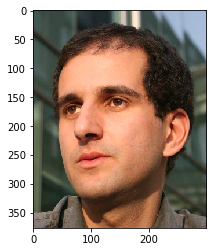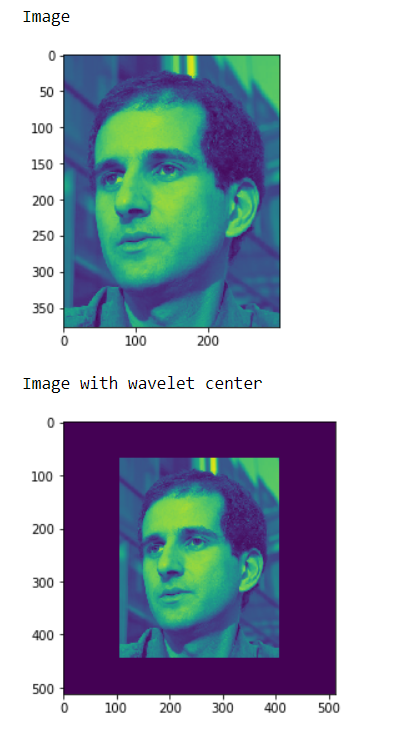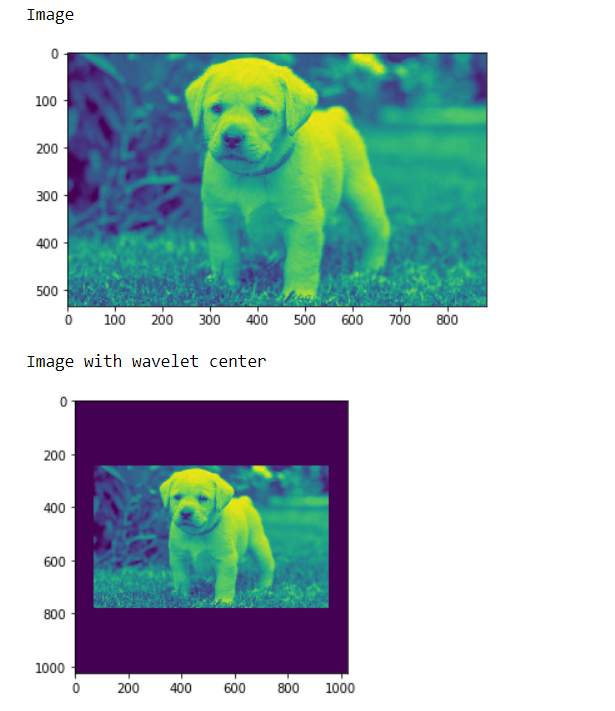# Mahotas – Making Image Wavelet Center

In this article we will see how we can make the image wavelet center in mahotas. Wavelets represent the scale of features in an image, as well as their position. Wavelet center will make the image small and place it in the center unlike daubechies wavelet.

In this tutorial we will use “luispedro” image, below is the command to load it.

`mahotas.demos.load('luispedro')`

Below is the luispedro imageIn order to do this we will use `wavelet_center` method

Syntax : mahotas.wavelet_center(img)

Argument : It takes image object as argument

Return : It returns image object

Note : Input image should be filtered or should be loaded as grey

In order to filter the image we will take the image object which is numpy.ndarray and filter it with the help of indexing, below is the command to do this

`image = image[:, :, 0]`

Example 1:

 `# importing various libraries ` `import` `numpy as np ` `import` `mahotas ` `import` `mahotas.demos ` `from` `mahotas.thresholding ``import` `soft_threshold ` `from` `pylab ``import` `imshow, show ` `from` `os ``import` `path ` ` `  `# loading image ` `f ``=` `mahotas.demos.load(``'luispedro'``, as_grey ``=` `True``) ` ` `  ` `  `# showing image ` `print``(``"Image"``) ` ` `  `imshow(f) ` `show() ` ` `  `# making image wavelet center ` `fc ``=` `mahotas.wavelet_center(f) ` ` `  `# showing image ` `print``(``"Image with wavelet center"``) ` `imshow(fc) ` `show() `

Output :Example 2:

 `# importing required libraries ` `import` `mahotas ` `import` `numpy as np ` `from` `pylab ``import` `imshow, show ` `import` `os ` ` `  ` `  `# loading iamge ` `img ``=` `mahotas.imread(``'dog_image.png'``) ` ` `  `# filtering iamge ` `img ``=` `img[:, :, ``0``] ` ` `  ` `  `# showing image ` `print``(``"Image"``) ` ` `  `imshow(img) ` `show() ` ` `  `# making image wavelet center ` `fc ``=` `mahotas.wavelet_center(img) ` ` `  `# showing image ` `print``(``"Image with wavelet center"``) ` `imshow(fc) ` `show() `

Output :My Personal Notes arrow_drop_upCheck out this Author's contributed articles.

If you like GeeksforGeeks and would like to contribute, you can also write an article using contribute.geeksforgeeks.org or mail your article to contribute@geeksforgeeks.org. See your article appearing on the GeeksforGeeks main page and help other Geeks.

Please Improve this article if you find anything incorrect by clicking on the "Improve Article" button below.

Article Tags :

Be the First to upvote.

Please write to us at contribute@geeksforgeeks.org to report any issue with the above content.